# 40 Stoichiometry of Gases

### Learning Objectives

By the end of this section, you will be able to:

• Use the ideal gas law to compute gas densities and molar masses
• Perform stoichiometric calculations involving gaseous substances
• State Dalton’s law of partial pressures and use it in calculations involving gaseous mixtures

The study of the chemical behavior of gases was part of the basis of perhaps the most fundamental chemical revolution in history. French nobleman Antoine Lavoisier, widely regarded as the “father of modern chemistry,” changed chemistry from a qualitative to a quantitative science through his work with gases. He discovered the law of conservation of matter, discovered the role of oxygen in combustion reactions, determined the composition of air, explained respiration in terms of chemical reactions, and more. He was a casualty of the French Revolution, guillotined in 1794. Of his death, mathematician and astronomer Joseph-Louis Lagrange said, “It took the mob only a moment to remove his head; a century will not suffice to reproduce it.”

As described in an earlier chapter of this text, we can turn to chemical stoichiometry for answers to many of the questions that ask “How much?” We can answer the question with masses of substances or volumes of solutions. However, we can also answer this question another way: with volumes of gases. We can use the ideal gas equation to relate the pressure, volume, temperature, and number of moles of a gas. Here we will combine the ideal gas equation with other equations to find gas density and molar mass. We will deal with mixtures of different gases, and calculate amounts of substances in reactions involving gases. This section will not introduce any new material or ideas, but will provide examples of applications and ways to integrate concepts we have already discussed.

## Density of a Gas

Recall that the density of a gas is its mass to volume ratio, $\rho =\frac{m}{V}.$ Therefore, if we can determine the mass of some volume of a gas, we will get its density. The density of an unknown gas can used to determine its molar mass and thereby assist in its identification. The ideal gas law, PV = nRT, provides us with a means of deriving such a mathematical formula to relate the density of a gas to its volume in the proof shown in Example 1.

### Example 1: Derivation of a Density Formula from the Ideal Gas Law

Use PV = nRT to derive a formula for the density of gas in g/L.

• PV = nRT
• Rearrange to get (mol/L): $\frac{n}{v}=\frac{P}{RT}$
• Multiply each side of the equation by the molar mass, ℳ. When moles are multiplied by ℳ in g/mol, g are obtained:
$\left(\mathcal{M}\right)\left(\frac{n}{V}\right)=\left(\frac{P}{RT}\right)\left(\mathcal{M}\right)$
• $g\text{/L}=\rho =\frac{P\mathcal{M}}{RT}$

A gas was found to have a density of 0.0847 g/L at 17.0 °C and a pressure of 760 torr. What is its molar mass? What is the gas?

$\rho =\frac{P\mathcal{M}}{RT}$

$0.0847\text{g/L}=760\cancel{\text{torr}}\times \frac{1\cancel{\text{atm}}}{760\cancel{\text{torr}}}\times \frac{\mathcal{M}}{\text{0.0821 L}\cancel{\text{atm}}\text{/mol K}}\times \text{290 K}$

ℳ = 2.02 g/mol; therefore, the gas must be hydrogen (H2, 2.02 g/mol)

We must specify both the temperature and the pressure of a gas when calculating its density, because the number of moles of a gas (and thus the mass of the gas) in a liter changes with temperature or pressure. Gas densities are often reported at STP.

### Example 2: Empirical/Molecular Formula Problems Using the Ideal Gas Law and Density of a Gas

Cyclopropane, a gas once used with oxygen as a general anesthetic, is composed of 85.7% carbon and 14.3% hydrogen by mass. Find the empirical formula. If 1.56 g of cyclopropane occupies a volume of 1.00 L at 0.984 atm and 50 °C, what is the molecular formula for cyclopropane?

Strategy: First solve the empirical formula problem using methods discussed earlier. Assume 100 g and convert the percentage of each element into grams. Determine the number of moles of carbon and hydrogen in the 100-g sample of cyclopropane. Divide by the smallest number of moles to relate the number of moles of carbon to the number of moles of hydrogen. In the last step, realize that the smallest whole number ratio is the empirical formula:

$\text{85.7 g C}\times \frac{\text{1 mol C}}{\text{12.01 g C}}=\text{7.136 mol C}\frac{7.136}{7.136}=\text{1.00 mol C}$
$\text{14.3 g H}\times \frac{\text{1 mol H}}{\text{1.01 g H}}=\text{14.158 mol H}\frac{14.158}{7.136}=\text{1.98 mol H}$

Empirical formula is CH2 [empirical mass (EM) of 14.03 g/empirical unit].

Next, use the density equation related to the ideal gas law to determine the molar mass:

$\text{d}=\frac{\text{P}\mathcal{M}}{\text{RT}}\frac{\text{1.56 g}}{\text{1.00 L}}=\text{0.984 atm}\times \frac{\mathcal{M}}{\text{0.0821 L atm/mol K}}\times \text{323 K}$

ℳ = 42.0 g/mol, $\frac{\mathcal{M}}{\text{E}\mathcal{M}}=\frac{42.0}{14.03}=2.99,$ so (3)(CH2) = C3H6 (molecular formula).

Acetylene, a fuel used welding torches, is comprised of 92.3% C and 7.7% H by mass. Find the empirical formula. If g of acetylene occupies of volume of 1.00 L at 1.15 atm and 59.5 °C, what is the molecular formula for acetylene?

Empirical formula, CH; Molecular formula, C2H2

## Molar Mass of a Gas

Another useful application of the ideal gas law involves the determination of molar mass. By definition, the molar mass of a substance is the ratio of its mass in grams, m, to its amount in moles, n

$\mathcal{M}=\frac{\text{grams of substance}}{\text{moles of substance}}=\frac{m}{n}$

The ideal gas equation can be rearranged to isolate n:

$n=\frac{PV}{RT}$

and then combined with the molar mass equation to yield:

$\mathcal{M}=\frac{mRT}{PV}$

This equation can be used to derive the molar mass of a gas from measurements of its pressure, volume, temperature, and mass.

### Example 3: Determining the Molar Mass of a Volatile Liquid

The approximate molar mass of a volatile liquid can be determined by:

1. Heating a sample of the liquid in a flask with a tiny hole at the top, which converts the liquid into gas that may escape through the hole
2. Removing the flask from heat at the instant when the last bit of liquid becomes gas, at which time the flask will be filled with only gaseous sample at ambient pressure
3. Sealing the flask and permitting the gaseous sample to condense to liquid, and then weighing the flask to determine the sample’s mass (see Figure 1)

Using this procedure, a sample of chloroform gas weighing 0.494 g is collected in a flask with a volume of 129 cm3 at 99.6 °C when the atmospheric pressure is 742.1 mm Hg. What is the approximate molar mass of chloroform?

Since $\mathcal{M}=\frac{m}{n}$ and $n=\frac{PV}{RT},$ substituting and rearranging gives $\mathcal{M}=\frac{mRT}{PV},$ then

$\mathcal{M}=\frac{mRT}{PV}=\frac{\left(\text{0.494 g}\right)\times \text{0.08206 L\cdot atm/mol K}\times \text{372.8 K}}{\text{0.976 atm}\times \text{0.129 L}}=120\text{ g/mol}$

A sample of phosphorus that weighs 3.243 × 10-2 g exerts a pressure of 31.89 kPa in a 56.0-mL bulb at 550 °C. What are the molar mass and molecular formula of phosphorus vapor?

124 g/mol P4

## The Pressure of a Mixture of Gases: Dalton’s Law

Unless they chemically react with each other, the individual gases in a mixture of gases do not affect each other’s pressure. Each individual gas in a mixture exerts the same pressure that it would exert if it present alone in the container (Figure 2). The pressure exerted by each individual gas in a mixture is called its partial pressure. This observation is summarized by Dalton’s law of partial pressures: The total pressure of a mixture of ideal gases is equal to the sum of the partial pressures of the component gases:

${P}_{Total}={P}_{A}+{P}_{B}+{P}_{C}+\ldots ={\Sigma}_{\text{i}}{P}_{\text{i}}$

In the equation PTotal is the total pressure of a mixture of gases, PA is the partial pressure of gas A; PB is the partial pressure of gas B; PC is the partial pressure of gas C; and so on.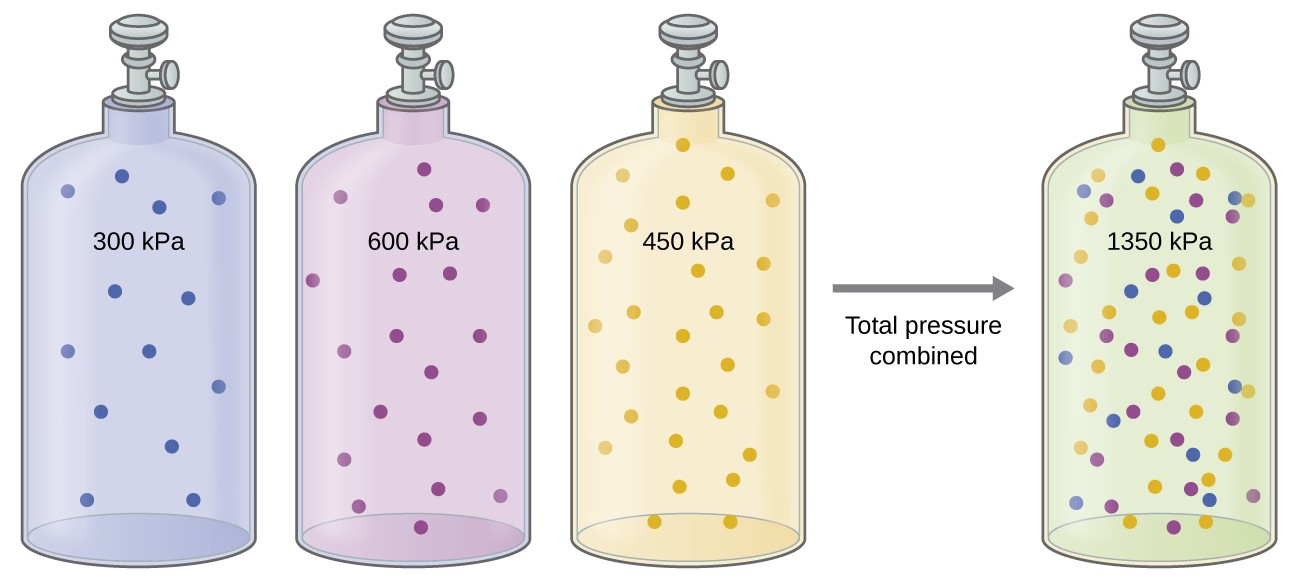Figure 2. If equal-volume cylinders containing gas A at a pressure of 300 kPa, gas B at a pressure of 600 kPa, and gas C at a pressure of 450 kPa are all combined in the same-size cylinder, the total pressure of the mixture is 1350 kPa.

The partial pressure of gas A is related to the total pressure of the gas mixture via its mole fraction, a unit of concentration defined as the number of moles of a component of a solution divided by the total number of moles of all components):

${P}_{A}={X}_{A}\times {P}_{Total}\text{ where }{X}_{A}=\frac{{n}_{A}}{{n}_{Total}}$

where PA, XA, and nA are the partial pressure, mole fraction, and number of moles of gas A, respectively, and nTotal is the number of moles of all components in the mixture.

### Example 4: The Pressure of a Mixture of Gases

A 10.0-L vessel contains 2.50 × 10-3 mol of H2, 1.00 × 10-3 mol of He, and 3.00 × 10-4 mol of Ne at 35 °C.

1. What are the partial pressures of each of the gases?
2. What is the total pressure in atmospheres?

The gases behave independently, so the partial pressure of each gas can be determined from the ideal gas equation, using $P=\frac{nRT}{V}:$

${P}_{{\text{H}}_{2}}=\frac{\left(2.50\times {10}^{-\text{3}}\cancel{\text{mol}}\right)\left(0.08206\cancel{\text{L}}\text{atm}\cancel{{\text{mol}}^{-\text{1}}{\text{K}}^{-\text{1}}}\right)\left(\text{308 K}\right)}{10.0\cancel{\text{L}}}=6.32\times {10}^{-\text{3}}\text{atm}$

${P}_{\text{He}}=\frac{\left(1.00\times {10}^{-\text{3}}\cancel{\text{mol}}\right)\left(\text{0.08206 L atm}\cancel{{\text{mol}}^{-\text{1}}{\text{K}}^{-\text{1}}}\right)\left(\text{308 K}\right)}{10.0\cancel{\text{L}}}=2.53\times {10}^{-\text{3}}\text{atm}$

${P}_{\text{Ne}}=\frac{\left(3.00\times {10}^{-\text{4}}\cancel{\text{mol}}\right)\left(0.08206\cancel{\text{L}}\text{atm}\cancel{{\text{mol}}^{-\text{1}}{\text{K}}^{-\text{1}}}\right)\left(\text{308 K}\right)}{10.0\cancel{\text{L}}}=7.58\times {10}^{-\text{4}}\text{atm}$

The total pressure is given by the sum of the partial pressures:

${P}_{\text{T}}={P}_{{\text{H}}_{2}}+{P}_{\text{He}}+{P}_{\text{Ne}}=\left(0.00632+0.00253+0.00076\right)\text{atm}=9.61\times {10}^{-\text{3}}\text{atm}$

A 5.73-L flask at 25 °C contains 0.0388 mol of N2, 0.147 mol of CO, and 0.0803 mol of H2. What is the total pressure in the flask in atmospheres?

1.137 atm

Here is another example of this concept, but dealing with mole fraction calculations.

### Example 5: The Pressure of a Mixture of Gases

A gas mixture used for anesthesia contains 2.83 mol oxygen, O2, and 8.41 mol nitrous oxide, N2O. The total pressure of the mixture is 192 kPa.

1. What are the mole fractions of O2 and N2O?
2. What are the partial pressures of O2 and N2O?

The mole fraction is given by ${X}_{A}=\frac{{n}_{A}}{{n}_{Total}}$ and the partial pressure is PA = XA × PTotal.

For O2,

${X}_{{O}_{2}}=\frac{{n}_{{O}_{2}}}{{n}_{Total}}=\frac{\text{2.83 mol}}{\left(2.83+8.41\right)\text{mol}}=0.252$

and

${P}_{{O}_{2}}={X}_{{O}_{2}}\times {P}_{Total}=0.252\times \text{192 kPa}=\text{48.4 kPa}$

For N2O,

${X}_{{O}_{2}}=\frac{{n}_{{O}_{2}}}{{n}_{Total}}=\frac{\text{2.83 mol}}{\left(2.83+8.41\right)\text{mol}}=0.252$

and

${P}_{{O}_{2}}={X}_{{O}_{2}}\times {P}_{Total}=\left(0.252\right)\times \text{192 kPa}$

${P}_{{O}_{2}}={X}_{{O}_{2}}\times {P}_{Total}=0.252\times \text{192 kPa}=\text{48.4 kPa}$

What is the pressure of a mixture of 0.200 g of H2, 1.00 g of N2, and 0.820 g of Ar in a container with a volume of 2.00 L at 20 °C?

1.87 atm

## Collection of Gases over Water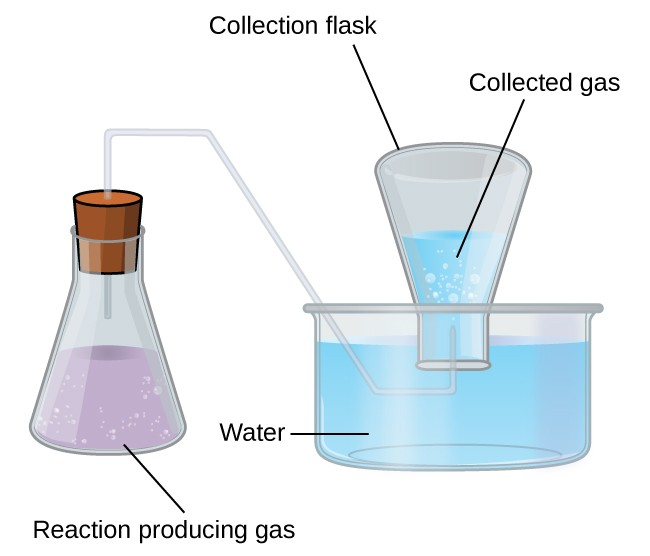Figure 3. When a reaction produces a gas that is collected above water, the trapped gas is a mixture of the gas produced by the reaction and water vapor. If the collection flask is appropriately positioned to equalize the water levels both within and outside the flask, the pressure of the trapped gas mixture will equal the atmospheric pressure outside the flask (see the earlier discussion of manometers).

A simple way to collect gases that do not react with water is to capture them in a bottle that has been filled with water and inverted into a dish filled with water. The pressure of the gas inside the bottle can be made equal to the air pressure outside by raising or lowering the bottle. When the water level is the same both inside and outside the bottle (Figure 3), the pressure of the gas is equal to the atmospheric pressure, which can be measured with a barometer.

However, there is another factor we must consider when we measure the pressure of the gas by this method. Water evaporates and there is always gaseous water (water vapor) above a sample of liquid water. As a gas is collected over water, it becomes saturated with water vapor and the total pressure of the mixture equals the partial pressure of the gas plus the partial pressure of the water vapor. The pressure of the pure gas is therefore equal to the total pressure minus the pressure of the water vapor—this is referred to as the “dry” gas pressure, that is, the pressure of the gas only, without water vapor. The vapor pressure of water, which is the pressure exerted by water vapor in equilibrium with liquid water in a closed container, depends on the temperature (Figure 4); more detailed information on the temperature dependence of water vapor can be found in Table 1, and vapor pressure will be discussed in more detail in the next chapter on liquids.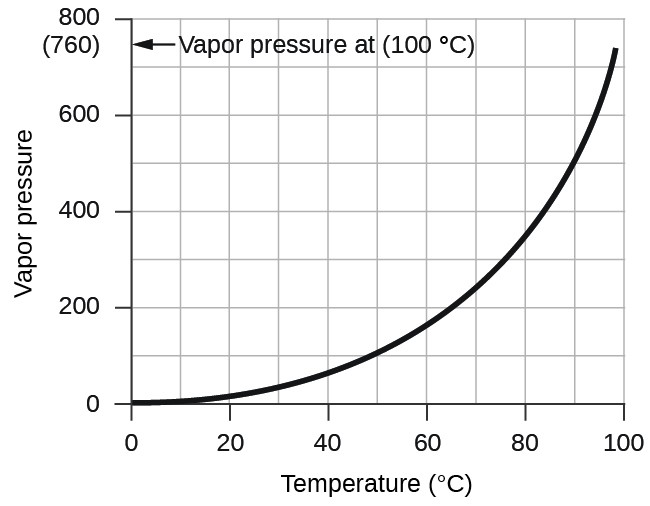Figure 4. This graph shows the vapor pressure of water at sea level as a function of temperature.
Table 1. Vapor Pressure of Ice and Water in Various Temperatures at Sea Level
Temperature (°C) Pressure (torr) Temperature (°C) Pressure (torr) Temperature (°C) Pressure (torr)
–10 1.95 18 15.5 30 31.8
–5 3.0 19 16.5 35 42.2
–2 3.9 20 17.5 40 55.3
0 4.6 21 18.7 50 92.5
2 5.3 22 19.8 60 149.4
4 6.1 23 21.1 70 233.7
6 7.0 24 22.4 80 355.1
8 8.0 25 23.8 90 525.8
10 9.2 26 25.2 95 633.9
12 10.5 27 26.7 99 733.2
14 12.0 28 28.3 100.0 760.0
16 13.6 29 30.0 101.0 787.6

### Example 6: Pressure of a Gas Collected Over Water

If 0.200 L of argon is collected over water at a temperature of 26 °C and a pressure of 750 torr in a system like that shown in Figure 3, what is the partial pressure of argon?

According to Dalton’s law, the total pressure in the bottle (750 torr) is the sum of the partial pressure of argon and the partial pressure of gaseous water:

${P}_{\text{T}}={P}_{\text{Ar}}+{P}_{{\text{H}}_{2}\text{O}}$

Rearranging this equation to solve for the pressure of argon gives:

${P}_{\text{Ar}}={P}_{\text{T}}-{P}_{{\text{H}}_{2}\text{O}}$

The pressure of water vapor above a sample of liquid water at 26 °C is 25.2 torr (Water Properties), so:

${P}_{\text{Ar}}=750\text{ torr}-25.2\text{ torr}=725\text{ torr}$

A sample of oxygen collected over water at a temperature of 29.0 °C and a pressure of 764 torr has a volume of 0.560 L. What volume would the dry oxygen have under the same conditions of temperature and pressure?

734 torr

## Chemical Stoichiometry and Gases

Chemical stoichiometry describes the quantitative relationships between reactants and products in chemical reactions.

We have previously measured quantities of reactants and products using masses for solids and volumes in conjunction with the molarity for solutions; now we can also use gas volumes to indicate quantities. If we know the volume, pressure, and temperature of a gas, we can use the ideal gas equation to calculate how many moles of the gas are present. If we know how many moles of a gas are involved, we can calculate the volume of a gas at any temperature and pressure.

Sometimes we can take advantage of a simplifying feature of the stoichiometry of gases that solids and solutions do not exhibit: All gases that show ideal behavior contain the same number of molecules in the same volume (at the same temperature and pressure). Thus, the ratios of volumes of gases involved in a chemical reaction are given by the coefficients in the equation for the reaction, provided that the gas volumes are measured at the same temperature and pressure.

We can extend Avogadro’s law (that the volume of a gas is directly proportional to the number of moles of the gas) to chemical reactions with gases: Gases combine, or react, in definite and simple proportions by volume, provided that all gas volumes are measured at the same temperature and pressure. For example, since nitrogen and hydrogen gases react to produce ammonia gas according to ${\text{N}}_{2}\left(g\right)+3{\text{H}}_{2}\left(g\right)\rightarrow{2{\text{NH}}}_{3}\left(g\right)$, a given volume of nitrogen gas reacts with three times that volume of hydrogen gas to produce two times that volume of ammonia gas, if pressure and temperature remain constant.

The explanation for this is illustrated in Figure 5. According to Avogadro’s law, equal volumes of gaseous N2, H2, and NH3, at the same temperature and pressure, contain the same number of molecules. Because one molecule of N2 reacts with three molecules of H2 to produce two molecules of NH3, the volume of H2 required is three times the volume of N2, and the volume of NH3 produced is two times the volume of N2.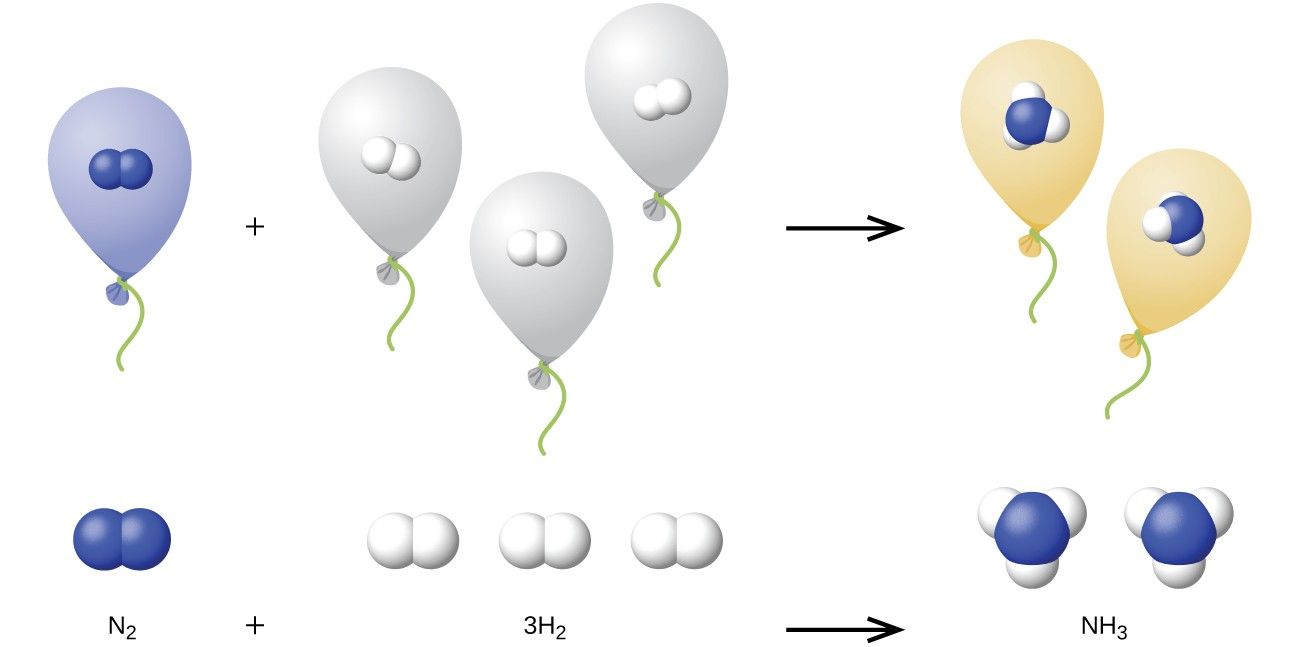Figure 5. One volume of N2 combines with three volumes of H2 to form two volumes of NH3.

### Example 7: Reaction of Gases

Propane, C3H8(g), is used in gas grills to provide the heat for cooking. What volume of O2(g) measured at 25 °C and 760 torr is required to react with 2.7 L of propane measured under the same conditions of temperature and pressure? Assume that the propane undergoes complete combustion.

The ratio of the volumes of C3H8 and O2 will be equal to the ratio of their coefficients in the balanced equation for the reaction:

$\begin{array}{ccccccc}{\text{C}}_{3}{\text{H}}_{8}\left(g\right)&+&5{\text{O}}_{2}\left(g\right)&\longrightarrow&3{\text{CO}}_{2}\left(g\right)&+&4{\text{H}}_{2}\text{O}\left(l\right)\\ \text{1 volume}&+&\text{5 volumes}&{}&\text{3 volumes}&+&\text{4 volumes}\end{array}$

From the equation, we see that one volume of C3H8 will react with five volumes of O2:

$2.7\cancel{\text{L}{\text{C}}_{3}{\text{H}}_{8}}\times \frac{\text{5 L}{\text{O}}_{2}}{1\cancel{\text{L}{\text{C}}_{3}{\text{H}}_{8}}}=\text{13.5 L}{\text{O}}_{2}$

A volume of 13.5 L of O2 will be required to react with 2.7 L of C3H8.

An acetylene tank for an oxyacetylene welding torch provides 9340 L of acetylene gas, C2H2, at 0°C and 1 atm. How many tanks of oxygen, each providing 7.00 × 103 L of O2 at 0 °C and 1 atm, will be required to burn the acetylene?

$2{\text{C}}_{2}{\text{H}}_{2}+5{\text{O}}_{2}\rightarrow 4{\text{CO}}_{2}+2{\text{H}}_{2}\text{O}$

3.34 tanks (2.34 × 104 L)

### Example 8: Volumes of Reacting Gases

Ammonia is an important fertilizer and industrial chemical. Suppose that a volume of 683 billion cubic feet of gaseous ammonia, measured at 25 °C and 1 atm, was manufactured. What volume of H2(g), measured under the same conditions, was required to prepare this amount of ammonia by reaction with N2?

${\text{N}}_{2}\left(g\right)+3{\text{H}}_{2}\left(g\right)\rightarrow 2{\text{NH}}_{3}\left(g\right)$

Because equal volumes of H2 and NH3 contain equal numbers of molecules and each three molecules of H2 that react produce two molecules of NH3, the ratio of the volumes of H2 and NH3 will be equal to 3:2. Two volumes of NH3, in this case in units of billion ft3, will be formed from three volumes of H2:

$683\cancel{\text{ billion}{\text{ ft}}^{3}{\text{NH}}_{3}}\times \frac{\text{3 billion}{\text{ ft}}^{3}{\text{H}}_{2}}{2\cancel{\text{ billion}{\text{ ft}}^{3}{\text{NH}}_{3}}}=1.02\times {10}^{3}\text{billion}{\text{ ft}}^{3}{\text{H}}_{2}$

The manufacture of 683 billion ft3 of NH3 required 1020 billion ft3 of H2. (At 25 °C and 1 atm, this is the volume of a cube with an edge length of approximately 1.9 miles.)

What volume of O2(g) measured at 25 °C and 760 torr is required to react with 17.0 L of ethylene, C2H4(g), measured under the same conditions of temperature and pressure? The products are CO2 and water vapor.

51.0 L

### Example 9: Volume of Gaseous Product

What volume of hydrogen at 27 °C and 723 torr may be prepared by the reaction of 8.88 g of gallium with an excess of hydrochloric acid?

$2\text{Ga}\left(s\right)+6\text{HCl}\left(aq\right)\rightarrow 2{\text{GaCl}}_{3}\left(aq\right)+3{\text{H}}_{2}\left(g\right)$

To convert from the mass of gallium to the volume of H2(g), we need to do something like this: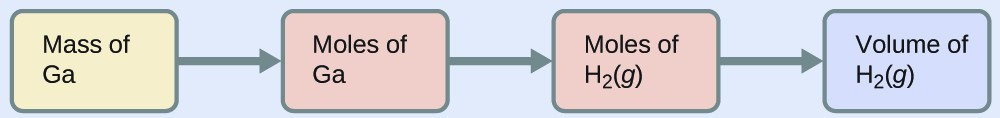The first two conversions are:

$8.88\cancel{\text{g Ga}}\times \frac{1\cancel{\text{mol Ga}}}{69.723\cancel{\text{g Ga}}}\times \frac{\text{3 mol}{\text{H}}_{2}}{2\cancel{\text{mol Ga}}}=0.191{\text{mol H}}_{2}$

Finally, we can use the ideal gas law:

${V}_{{\text{H}}_{2}}={\left(\frac{nRT}{P}\right)}_{{\text{H}}_{2}}=\frac{0.191\cancel{\text{mol}}\times \text{0.08206 L}\cancel{\text{atm}{\text{mol}}^{-\text{1}}{\text{K}}^{-\text{1}}}\times \text{300 K}}{0.951\cancel{\text{atm}}}=\text{4.94 L}$

Sulfur dioxide is an intermediate in the preparation of sulfuric acid. What volume of SO2 at 343 °C and 1.21 atm is produced by burning l.00 kg of sulfur in oxygen?

1.30 × 103 L

### Greenhouse Gases and Climate Change

The thin skin of our atmosphere keeps the earth from being an ice planet and makes it habitable. In fact, this is due to less than 0.5% of the air molecules. Of the energy from the sun that reaches the earth, almost $\frac{1}{3}$ is reflected back into space, with the rest absorbed by the atmosphere and the surface of the earth. Some of the energy that the earth absorbs is re-emitted as infrared (IR) radiation, a portion of which passes back out through the atmosphere into space. However, most of this IR radiation is absorbed by certain substances in the atmosphere, known as greenhouse gases, which re-emit this energy in all directions, trapping some of the heat. This maintains favorable living conditions—without atmosphere, the average global average temperature of 14 °C (57 °F) would be about –19 °C (–2 °F). The major greenhouse gases (GHGs) are water vapor, carbon dioxide, methane, and ozone. Since the Industrial Revolution, human activity has been increasing the concentrations of GHGs, which have changed the energy balance and are significantly altering the earth’s climate (Figure 6).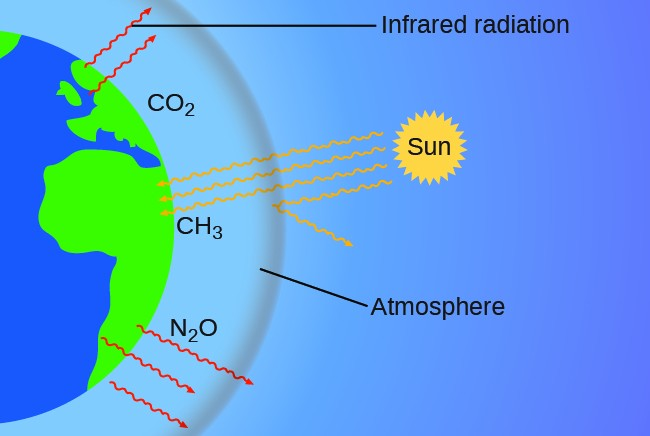Figure 6. Greenhouse gases trap enough of the sun’s energy to make the planet habitable—this is known as the greenhouse effect. Human activities are increasing greenhouse gas levels, warming the planet and causing more extreme weather events.

There is strong evidence from multiple sources that higher atmospheric levels of CO2 are caused by human activity, with fossil fuel burning accounting for about $\frac{3}{4}$ of the recent increase in CO2. Reliable data from ice cores reveals that CO2 concentration in the atmosphere is at the highest level in the past 800,000 years; other evidence indicates that it may be at its highest level in 20 million years. In recent years, the CO2 concentration has increased from historical levels of below 300 ppm to almost 400 ppm today (Figure 7).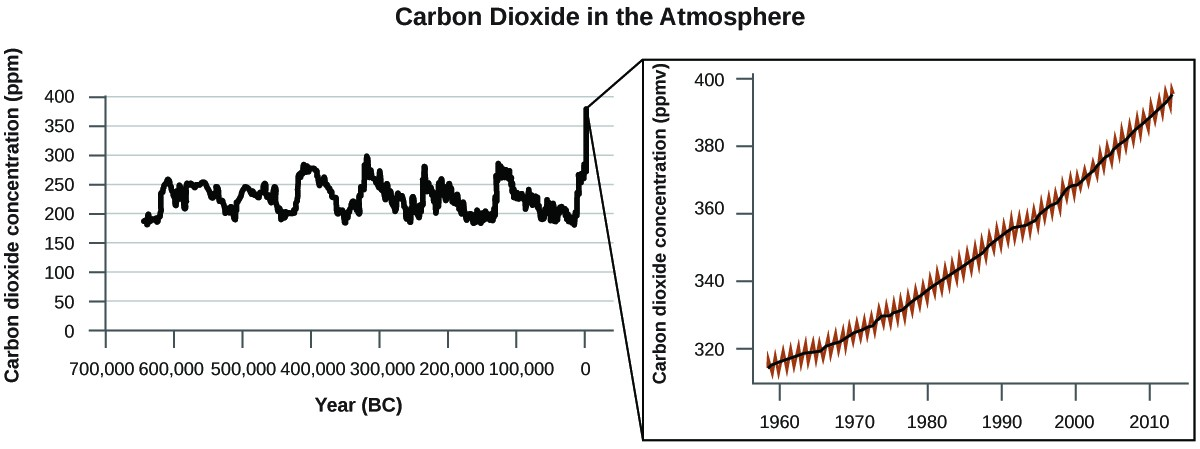### Portrait of a Chemist: Susan Solomon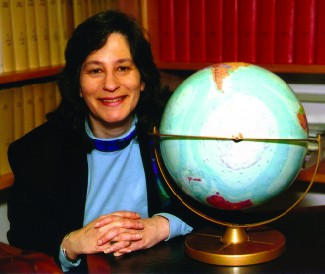Figure 8. Susan Solomon’s research focuses on climate change and has been instrumental in determining the cause of the ozone hole over Antarctica. (credit: National Oceanic and Atmospheric Administration)

Atmospheric and climate scientist Susan Solomon (Figure 8) is the author of one of The New York Times books of the year (The Coldest March, 2001), one of Time magazine’s 100 most influential people in the world (2008), and a working group leader of the Intergovernmental Panel on Climate Change (IPCC), which was the recipient of the 2007 Nobel Peace Prize. She helped determine and explain the cause of the formation of the ozone hole over Antarctica, and has authored many important papers on climate change. She has been awarded the top scientific honors in the US and France (the National Medal of Science and the Grande Medaille, respectively), and is a member of the National Academy of Sciences, the Royal Society, the French Academy of Sciences, and the European Academy of Sciences. Formerly a professor at the University of Colorado, she is now at MIT, and continues to work at NOAA.

### Key Concepts and Summary

The ideal gas law can be used to derive a number of convenient equations relating directly measured quantities to properties of interest for gaseous substances and mixtures. Appropriate rearrangement of the ideal gas equation may be made to permit the calculation of gas densities and molar masses. Dalton’s law of partial pressures may be used to relate measured gas pressures for gaseous mixtures to their compositions. Avogadro’s law may be used in stoichiometric computations for chemical reactions involving gaseous reactants or products.

#### Key Equations

• PTotal = PA + PB + PC + … = ƩiPi
• PA = XA PTotal
• ${X}_{A}=\frac{{n}_{A}}{{n}_{Total}}$

### Exercises

1. What is the density of laughing gas, dinitrogen monoxide, N2O, at a temperature of 325 K and a pressure of 113.0 kPa?
2. Calculate the density of Freon 12, CF2Cl2, at 30.0°C and 0.954 atm.
3. Which is denser at the same temperature and pressure, dry air or air saturated with water vapor? Explain.
4. A cylinder of O2(g) used in breathing by emphysema patients has a volume of 3.00 L at a pressure of 10.0 atm. If the temperature of the cylinder is 28.0 °C, what mass of oxygen is in the cylinder?
5. What is the molar mass of a gas if 0.0494 g of the gas occupies a volume of 0.100 L at a temperature 26 °C and a pressure of 307 torr?
6. What is the molar mass of a gas if 0.281 g of the gas occupies a volume of 125 mL at a temperature 126 °C and a pressure of 777 torr?
7. How could you show experimentally that the molecular formula of propene is C3H6, not CH2?
8. The density of a certain gaseous fluoride of phosphorus is 3.93 g/L at STP. Calculate the molar mass of this fluoride and determine its molecular formula.
9. Consider this question: What is the molecular formula of a compound that contains 39% C, 45% N, and 16% H if 0.157 g of the compound occupies l25 mL with a pressure of 99.5 kPa at 22 °C?
1. Outline the steps necessary to answer the question.
10. A 36.0–L cylinder of a gas used for calibration of blood gas analyzers in medical laboratories contains 350 g CO2, 805 g O2, and 4,880 g N2. What is the pressure in the flask in atmospheres, in torr, and in kilopascals?
11. A cylinder of a gas mixture used for calibration of blood gas analyzers in medical laboratories contains 5.0% CO2, 12.0% O2, and the remainder N2 at a total pressure of 146 atm. What is the partial pressure of each component of this gas? (The percentages given indicate the percent of the total pressure that is due to each component.)
12. A sample of gas isolated from unrefined petroleum contains 90.0% CH4, 8.9% C2H6, and 1.1% C3H8 at a total pressure of 307.2 kPa. What is the partial pressure of each component of this gas? (The percentages given indicate the percent of the total pressure that is due to each component.)
13. A mixture of 0.200 g of H2, 1.00 g of N2, and 0.820 g of Ar is stored in a closed container at STP. Find the volume of the container, assuming that the gases exhibit ideal behavior.
14. Most mixtures of hydrogen gas with oxygen gas are explosive. However, a mixture that contains less than 3.0 % O2 is not. If enough O2 is added to a cylinder of H2 at 33.2 atm to bring the total pressure to 34.5 atm, is the mixture explosive?
15. A commercial mercury vapor analyzer can detect, in air, concentrations of gaseous Hg atoms (which are poisonous) as low as 2 × 10-6 mg/L of air. At this concentration, what is the partial pressure of gaseous mercury if the atmospheric pressure is 733 torr at 26 °C?
16. A sample of carbon monoxide was collected over water at a total pressure of 756 torr and a temperature of 18 °C. What is the pressure of the carbon monoxide? (See Table 9.2 for the vapor pressure of water.)
17. In an experiment in a general chemistry laboratory, a student collected a sample of a gas over water. The volume of the gas was 265 mL at a pressure of 753 torr and a temperature of 27 °C. The mass of the gas was 0.472 g. What was the molar mass of the gas?
18. Joseph Priestley first prepared pure oxygen by heating mercuric oxide, HgO:  $2\text{HgO}\left(s\right)\rightarrow 2\text{Hg}\left(l\right)+{\text{O}}_{2}\left(g\right)$
1. Outline the steps necessary to answer the following question: What volume of O2 at 23° C and 0.975 atm is produced by the decomposition of 5.36 g of HgO?
19. Cavendish prepared hydrogen in 1766 by the novel method of passing steam through a red-hot gun barrel:$4{\text{H}}_{2}\text{O}\left(g\right)+3\text{Fe}\left(s\right)\rightarrow{\text{Fe}}_{3}{\text{O}}_{4}\left(s\right)+4{\text{H}}_{2}\left(g\right)$
1. Outline the steps necessary to answer the following question: What volume of H2 at a pressure of 745 torr and a temperature of 20 °C can be prepared from the reaction of 15.O g of H2O?
20. The chlorofluorocarbon CCl2F2 can be recycled into a different compound by reaction with hydrogen to produce CH2F2(g), a compound useful in chemical manufacturing: ${\text{CCl}}_{2}{\text{F}}_{2}\left(g\right)+4{\text{H}}_{2}\left(g\right)\rightarrow{\text{CH}}_{2}{\text{F}}_{2}\left(g\right)+2\text{HCl}\left(g\right).$
1. Outline the steps necessary to answer the following question: What volume of hydrogen at 225 atm and 35.5 °C would be required to react with 1 ton (1.000 × 103 kg) of CCl2F2?
21. Automobile air bags are inflated with nitrogen gas, which is formed by the decomposition of solid sodium azide (NaN3). The other product is sodium metal. Calculate the volume of nitrogen gas at 27 °C and 756 torr formed by the decomposition of 125 g of sodium azide.
22. Lime, CaO, is produced by heating calcium carbonate, CaCO3; carbon dioxide is the other product.
1. Outline the steps necessary to answer the following question: What volume of carbon dioxide at 875° and 0.966 atm is produced by the decomposition of 1 ton (1.000 × 103 kg) of calcium carbonate?
23. Before small batteries were available, carbide lamps were used for bicycle lights. Acetylene gas, C2H2, and solid calcium hydroxide were formed by the reaction of calcium carbide, CaC2, with water. The ignition of the acetylene gas provided the light. Currently, the same lamps are used by some cavers, and calcium carbide is used to produce acetylene for carbide cannons.
1. Outline the steps necessary to answer the following question: What volume of C2H2 at 1.005 atm and 12.2 °C is formed by the reaction of 15.48 g of CaC2 with water?
24. Calculate the volume of oxygen required to burn 12.00 L of ethane gas, C2H6, to produce carbon dioxide and water, if the volumes of C2H6 and O2 are measured under the same conditions of temperature and pressure.
25. What volume of O2 at STP is required to oxidize 8.0 L of NO at STP to NO2? What volume of NO2 is produced at STP?
26. Consider the following questions:
1. What is the total volume of the CO2(g) and H2O(g) at 600 °C and 0.888 atm produced by the combustion of 1.00 L of C2H6(g) measured at STP?
2. What is the partial pressure of H2O in the product gases?
27. Methanol, CH3OH, is produced industrially by the following reaction:
$\text{CO}\left(g\right)+2{\text{H}}_{2}\left(g\right)\,\,\,{\xrightarrow{\text{copper catalyst }300^{\circ}\text{ C}, 300\text{ atm}}}\,\,\,{\text{CH}}_{3}\text{OH}\left(g\right)$
Assuming that the gases behave as ideal gases, find the ratio of the total volume of the reactants to the final volume.
28. What volume of oxygen at 423.0 K and a pressure of 127.4 kPa is produced by the decomposition of 129.7 g of BaO2 to BaO and O2?
29. A 2.50-L sample of a colorless gas at STP decomposed to give 2.50 L of N2 and 1.25 L of O2 at STP. What is the colorless gas?
30. Ethanol, C2H5OH, is produced industrially from ethylene, C2H4, by the following sequence of reactions:
$3{\text{C}}_{2}{\text{H}}_{4}+2{\text{H}}_{2}{\text{SO}}_{4}\rightarrow{\text{C}}_{2}{\text{H}}_{5}{\text{HSO}}_{4}+{\left({\text{C}}_{2}{\text{H}}_{5}\right)}_{2}{\text{SO}}_{4}$ ${\text{C}}_{2}{\text{H}}_{5}{\text{HSO}}_{4}+{\left({\text{C}}_{2}{\text{H}}_{5}\right)}_{2}{\text{SO}}_{4}+3{\text{H}}_{2}\text{O}\rightarrow 3{\text{C}}_{2}{\text{H}}_{5}\text{OH}+2{\text{H}}_{2}{\text{SO}}_{4}$
What volume of ethylene at STP is required to produce 1.000 metric ton (1000 kg) of ethanol if the overall yield of ethanol is 90.1%?
31. One molecule of hemoglobin will combine with four molecules of oxygen. If 1.0 g of hemoglobin combines with 1.53 mL of oxygen at body temperature (37 °C) and a pressure of 743 torr, what is the molar mass of hemoglobin?
32. A sample of a compound of xenon and fluorine was confined in a bulb with a pressure of 18 torr. Hydrogen was added to the bulb until the pressure was 72 torr. Passage of an electric spark through the mixture produced Xe and HF. After the HF was removed by reaction with solid KOH, the final pressure of xenon and unreacted hydrogen in the bulb was 36 torr. What is the empirical formula of the xenon fluoride in the original sample? (Note: Xenon fluorides contain only one xenon atom per molecule.)
33. One method of analyzing amino acids is the van Slyke method. The characteristic amino groups (-NH2) in protein material are allowed to react with nitrous acid, HNO2, to form N2 gas. From the volume of the gas, the amount of amino acid can be determined. A 0.0604-g sample of a biological sample containing glycine, CH2(NH2)COOH, was analyzed by the van Slyke method and yielded 3.70 mL of N2 collected over water at a pressure of 735 torr and 29 °C. What was the percentage of glycine in the sample?
${\text{CH}}_{2}\left({\text{NH}}_{2}\right){\text{CO}}_{2}\text{H}+{\text{HNO}}_{2}\rightarrow{\text{CH}}_{2}\left(\text{OH}\right){\text{CO}}_{2}\text{H}+{\text{H}}_{2}\text{O}+{\text{N}}_{2}$

2. $\rho =\frac{P\mathcal{M}}{RT}=\frac{0.954\cancel{\text{atm}}\left[12.011+2\left(18.9954\right)+2\left(35.453\right)\right]\text{g}\cancel{{\text{mol}}^{-\text{1}}}}{\text{0.08206 L}\cancel{\text{atm}}\cancel{{\text{mol}}^{-\text{1}}}\cancel{{\text{K}}^{-\text{1}}}\times 303.15\cancel{\text{K}}}=\text{4.64 g}{\text{L}}^{-\text{1}}$

4. $\text{mass}{\text{O}}_{2}=\frac{\left(\text{31.9988 g}\cancel{{\text{mol}}^{-\text{1}}}\right)\left(10.0\cancel{\text{atm}}\right)\left(3.00\cancel{\text{L}}\right)}{\left(0.08206\cancel{\text{L}}\cancel{\text{atm}}\cancel{{\text{mol}}^{-\text{1}}}\cancel{{\text{K}}^{-\text{1}}}\right)\left(301.15\cancel{\text{K}}\right)}=\text{38.8 g}$

6. From the ideal gas law, PV = nRT, set $n=\frac{\text{mass}}{\text{molar mass}}$ and solve the molar mass. $\text{molar mass}=\frac{\left(\text{0.281 g}\right)\left(0.08206\cancel{\text{L}}\cancel{\text{atm}}{\text{mol}}^{-\text{1}}\cancel{{\text{K}}^{-\text{1}}}\right)\left(399.15\cancel{\text{K}}\right)}{\left(\frac{777\cancel{\text{torr}}}{760\cancel{\text{torr}}\cancel{{\text{atm}}^{-\text{1}}}}\right)\left(0.125\cancel{\text{L}}\right)}=\text{72.0 g}{\text{mol}}^{-\text{1}}$

8. $\mathcal{M}=\frac{mRT}{PV}D=\frac{m}{V}\mathcal{M}=\frac{DRT}{P}$ $\mathcal{M}=\frac{\text{3.93 g}{\text{L}}^{-\text{1}}\left(\text{0.08206 L}\cancel{\text{atm}}{\text{mol}}^{-\text{1}}\cancel{{\text{K}}^{-\text{1}}}\right)\left(273.15\cancel{\text{K}}\right)}{1.00\cancel{\text{atm}}}=\text{88.1 g}{\text{mol}}^{-\text{1}}$ ℳphosphorous = 30.97376 g/mol
fluorine = 18.998403 g/mol

$\begin{array}{rrl}\text{molecular formula:}&\text{ phosphorous: }&30.97376\\&{}\text{flourine:}&\underline{3\left(18.998403\right)}\\{}&{}&87.968969\end{array}$

The molecular formula is PF3.

To find this answer you can either use trial and error, or you can realize that since phosphorus is in group 5, it can fill its valence shell by forming three bonds. Fluorine, being in group 7, needs to form only one bond to fill its shell. Thus it makes sense to start with PF3 as a probable formula.

10. Calculate the moles of each gas present and from that, calculate the pressure from the ideal gas law. Assume 25°C. The calibration gas contains:

$\frac{350\cancel{\text{g}}{\text{CO}}_{2}}{44.0098\cancel{\text{g}}{\text{mol}}^{-\text{1}}{\text{CO}}_{2}}=\text{7.953 mol}{\text{ CO}}_{2}$
$\frac{805\cancel{\text{g}}{\text{O}}_{2}}{31.9988\cancel{\text{g}}{\text{mol}}^{-\text{1}}{\text{O}}_{2}}=\text{25.157 mol}{\text{ O}}_{2}$
$\frac{4880\cancel{\text{g}}{\text{N}}_{2}}{28.01348\cancel{\text{g}}{\text{mol}}^{-\text{1}}{\text{N}}_{2}}=\text{174.202 mol}{\text{ N}}_{2}$

Total moles = 7.953 + 25.157 + 174.202 = 207.312 mol

$P=\frac{nRT}{V}=\frac{207.312\cancel{\text{mol}}\times 0.08206\cancel{\text{L}}\text{atm}\cancel{{\text{mol}}^{-\text{1}}}\cancel{{\text{K}}^{-\text{1}}}\times 298.15\cancel{\text{K}}}{36.0\cancel{\text{L}}}=\text{141 atm}$

12. Since these are percentages of the total pressure, the partial pressure can be calculated as follows:

• CH4: 90% of 307.2 kPa = 0.900 × 307.2 = 276 kPa
• C2 H6: 8.9% of 307.2 kPa = 0.089 × 307.2 = 27 kPa
• C3 H8: 1.1% of 307.2 kPa = 0.011 × 307.2 = 3.4 kPa

14. The oxygen increases the pressure within the tank to (34.5 atm – 33.2 atm =) 1.3 atm. The percentage O2 on a mole basis is $\frac{1.3}{34.5}\times 100\%=3.77\%.$ The mixture is explosive. However, the percentage is given as a weight percent. Converting to a mass basis increases the percentage of oxygen even more, so the mixture is still explosive.

16. The vapor pressure of water at 18 °C is 15.5 torr. Subtract the vapor pressure of water from the total pressure to find the pressure of the carbon monoxide:

PT = Pgas + Pwater

Rearrangement gives: PT Pwater =Pgas

756 torr – 15.5 torr = 740 torr

18. The answers are as follows:

1. Determine the moles of HgO that decompose; using the chemical equation, determine the moles of O2 produced by decomposition of this amount of HgO; and determine the volume of O2 from the moles of O2, temperature, and pressure.
2. $\begin{array}{l}\\ \\ 5.36\cancel{\text{g HgO}}\times \frac{\text{1 mol HgO}}{\left(200.59+15.9994\right)\cancel{\text{g HgO}}}=\text{0.0247 mol HgO}\\ 0.0247\cancel{\text{mol HgO}}\times \frac{\text{1 mol}{\text{O}}_{2}}{2\cancel{\text{mol HgO}}}=\text{0.01235 mol}{\text{O}}_{2}\end{array}$

PV = nRT
P = 0.975 atm
T = (23.0 + 273.15) K

$V=\frac{nRT}{P}=\frac{0.01235\cancel{\text{mol}}\left(\text{0.08206 L}\cancel{\text{atm}}\cancel{{\text{mol}}^{-\text{1}}}\cancel{{\text{K}}^{-\text{1}}}\right)\left(296.15\cancel{\text{K}}\right)}{0.975\cancel{\text{atm}}}=0.308\text{ L}$

20. The answers are as follows:

1. Determine the molar mass of CCl2F2. From the balanced equation, calculate the moles of H2 needed for the complete reaction. From the ideal gas law, convert moles of H2 into volume.
2. Molar mass of CCl2 F2 = 12.011 + 2 × 18.9984 + 2 × 35.4527 = 120.913 g/mol
$\text{mol}{\text{H}}_{2}=1.000\times {10}^{6}\text{g}\times \frac{\text{1 mol}{\text{CCL}}_{2}{\text{F}}_{2}}{\text{120.913 g}}\times \frac{\text{4 mol}{\text{H}}_{2}}{\text{1 mol}{\text{CCl}}_{2}{\text{F}}_{2}}=3.308\times {10}^{4}\text{mol}$
$V=\frac{nRT}{P}=\frac{\left(3.308\times {10}^{4}\cancel{\text{mol}}\right)\left(\text{0.08206 L}\cancel{\text{atm}}\cancel{{\text{mol}}^{-\text{1}}}{\cancel{\text{K}}}^{-\text{1}}\right)\left(308.65\cancel{\text{K}}\right)}{225\cancel{\text{atm}}}=3.72\times {10}^{3}\text{L}$

22. The answers are as follows:

1. Balance the equation. Determine the grams of CO2 produced and the number of moles. From the ideal gas law, determine the volume of gas.
2. ${\text{CaCO}}_{3}\left(s\right)\rightarrow\text{CaO}\left(s\right)+{\text{CO}}_{2}\left(g\right)$
$\text{mass}{\text{CO}}_{2}=1.00\times {10}^{6}\text{g}\times \frac{\text{1 mol}{\text{CaCO}}_{2}}{\text{100.087 g}}\times \frac{\text{44.01 g}{\text{CO}}_{2}}{\text{1 mol}{\text{CO}}_{2}}\times \frac{\text{1 mol}{\text{CO}}_{2}}{\text{1 mol}{\text{CaCO}}_{2}}=4.397\times {10}^{5}\text{g}$
$\text{mol}{\text{CO}}_{2}=\frac{4.397\times {10}^{5}\text{g}}{\text{44.01 g}{\text{mol}}^{-\text{1}}}=\text{9991 mol}$
$V=\frac{nRT}{P}=\frac{\left(\text{9991 mol}\right)\left(\text{0.08206 L atm}{\text{mol}}^{-\text{1}}{\text{K}}^{-\text{1}}\right)\left(\text{875 K}\right)}{\text{0.966 atm}}=7.43\times {10}^{5}\text{L}$

24. $2{\text{C}}_{2}{\text{H}}_{6}\left(\text{g}\right)+7{\text{O}}_{2}\left(\text{g}\right)\rightarrow 4{\text{CO}}_{2}\left(\text{g}\right)+6{\text{H}}_{2}\text{O}\left(\text{g}\right)$

From the balanced equation, we see that 2 mol of C2H6 requires 7 mol of O2 to burn completely. Gay-Lussac’s law states that gases react in simple proportions by volume. As the number of liters is proportional to the number of moles,

$\frac{\text{12.00 L}}{\text{2 mol}{\text{C}}_{2}{\text{H}}_{6}}=\frac{V\left({\text{O}}_{2}\right)}{\text{7 mol}{\text{O}}_{2}}$
$V\left({\text{O}}_{2}\right)=\frac{\text{12.00 L}\times 7}{2}=\text{42.00 L}$

26. The answers are as follows:

(a) The scheme to solve this problem is:

${\text{volume C}}_{2}{\text{H}}_{6}\left(g\right)\stackrel{\begin{array}{l}\text{ideal gas}\\ \text{equation}\end{array}}{\to }{\text{mol C}}_{2}{\text{H}}_{6}\left(g\right)\stackrel{\begin{array}{l}\text{reaction}\\ \text{stoichiometry}\end{array}}{\to }{\text{mol CO}}_{2}+{\text{H}}_{2}\text{O}\stackrel{\begin{array}{l}\text{ideal gas}\\ \text{equation}\end{array}}{\to }{\text{volume CO}}_{2}+{\text{H}}_{2}\text{O}$
$\begin{array}{l}{\text{C}}_{2}{\text{H}}_{6}\left(g\right)+3\frac{1}{2}{\text{O}}_{2}\left(g\right)\rightarrow 2{\text{CO}}_{2}\left(g\right)+3{\text{H}}_{2}\text{O}\left(g\right)\\ \text{1}.n\left({\text{C}}_{2}{\text{H}}_{6}\right)=\frac{PV}{RT}=\frac{1.00\cancel{\text{atm}}\times 1.00\cancel{\text{L}}}{0.08206\cancel{\text{L}}\cancel{\text{atm}}{\text{mol}}^{-\text{1}}\cancel{{\text{K}}^{-\text{1}}}\left(273.15\cancel{\text{K}}\right)}=\text{0.0446 mol}\\ \text{2}.0.0446\cancel{\text{mol}{\text{C}}_{2}{\text{H}}_{6}}\times \frac{\text{5 mol products}}{1\cancel{\text{mol}{\text{C}}_{2}{\text{H}}_{6}}}=\text{0.223 mol products}\\ \text{3}.V=nRT=\frac{\left(0.223\cancel{\text{mol}}\right)\left(\text{0.08206 L}\cancel{\text{atm}}\cancel{{\text{mol}}^{-\text{1}}}\cancel{{\text{K}}^{-\text{1}}}\right)\left(873.15\cancel{\text{K}}\right)}{0.888\cancel{\text{atm}}}=\text{18.0 L}\end{array}$

(b) First, calculate the mol H2O produced:

$\text{0.0446 mol}{\text{C}}_{2}{\text{H}}_{6}\times \frac{\text{3 mol products}}{\text{1 mol}{\text{C}}_{2}{\text{H}}_{6}}=\text{0.1338 mol}$

Second, calculate the pressure of H2O:

$P=\frac{nRT}{V}=\frac{\left(0.1338\cancel{\text{mol}}\right)\left(0.8206\cancel{\text{L}}\text{atm}\cancel{{\text{mol}}^{-\text{1}}}\cancel{{\text{K}}^{-\text{1}}}\right)\left(873.15\cancel{\text{K}}\right)}{18.0\cancel{\text{L}}}=\text{0.533 atm}$

28. First, we must write a balanced equation to establish the stoichiometry of the reaction:

$2{\text{BaO}}_{2}\rightarrow 2\text{BaO}+{\text{O}}_{2}$
We are given the mass of BaO2 that decomposes, so the scheme for solving this problem will be: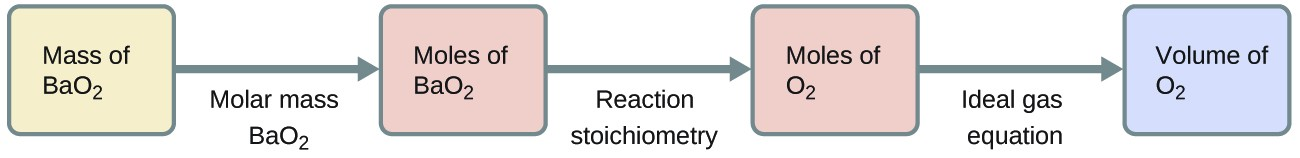Mass (BaO2) = 137.33 + 2(15.9994) = 169.33 g/mol

$n\left({\text{O}}_{2}\right)=\text{129.7 g}{\text{BaO}}_{2}\times \frac{\text{1 mol}{\text{BaO}}_{2}}{\text{169.33 g}{\text{BaO}}_{2}}\times \frac{\text{1 mol}{\text{O}}_{2}}{\text{2 mol}{\text{BaO}}_{2}}=\text{0.3830 mol}{\text{O}}_{2}$
$V\left({\text{O}}_{2}\right)=\frac{nRT}{P}=\frac{\text{0.3830 mol}\left(\text{8.314 L kPa}{\text{mol}}^{-\text{1}}{\text{K}}^{-\text{1}}\right)\left(\text{423.0 K}\right)}{\text{127.4 kPa}}=\text{10.57 L}{\text{O}}_{2}$

30. At 90.1% conversion, a 1.000 × 106 g final yield would require a $\left(\frac{1.000\times {10}^{6}}{0.901}\right)=1.1099\times {10}^{6}\text{g}$ theoretical yield.
3C2H4 produces 3C2H5OH, giving a 1:1 ratio:

$\text{mol}\left({\text{C}}_{2}{\text{H}}_{4}\right)=1.1099\times {10}^{6}\cancel{\text{g}{\text{C}}_{2}{\text{H}}_{5}\text{OH}}\times \frac{1\cancel{\text{mol}{\text{C}}_{2}{\text{H}}_{2}\text{OH}}}{46.069\cancel{\text{g}{\text{C}}_{2}{\text{H}}_{5}\text{OH}}}\times \frac{\text{1 mol}{\text{C}}_{2}{\text{H}}_{4}}{1\cancel{\text{mol}{\text{C}}_{2}{\text{H}}_{2}\text{OH}}}=2.409\times {10}^{4}\text{mol}$

V (C2 H4) = 22.4 L/mol × 2.409 × 104 mol = 5.40 × 105 L

32. The reaction is:

${\text{XeF}}_{x}+\frac{x}{2}{\text{H}}_{2}\rightarrow\text{Xe}+x\text{HF}$

The pressure of H2 that reacts is 48 torr – 24 torr = 24 torr

The number of moles of gas is proportional to the partial pressures. The reaction used 24 torr of XeFx and 24 torr of H2 so: $\frac{x}{2}=1$ and x = 2

The formula for the xenon compound is XeF2.

Immediately after the H2 is added (before the reaction):

$\begin{array}{lll} \hfill {P}_{\text{Total}}& =& {P}_{{\text{XeF}}_{2}}+{P}_{{\text{H}}_{2}}\hfill \\ \hfill {P}_{{\text{H}}_{2}}& =& {P}_{\text{Total}}-{P}_{{\text{XeF}}_{2}}\hfill \\ & =& \text{72 torr}-\text{24 torr}\hfill \\ & =& 48\text{torr}\hfill \end{array}$

After the reaction:

${P}_{\text{Xe}}=\text{24 torr}\text{ }\left(\text{1 mol}{\text{XeF}}_{\text{x}}\rightarrow\text{1 mol Xe}\right)$

And the partial pressure of unreacted H2 is:

$\begin{array}{ll}\hfill {P}_{{\text{H}}_{2}}& ={P}_{\text{Total}}-{P}_{\text{Xe}}\hfill \\ & =\text{48 torr}-\text{24 torr}\hfill \\ & =\text{24 torr}\hfill \end{array}$

## Glossary

Dalton’s law of partial pressures: total pressure of a mixture of ideal gases is equal to the sum of the partial pressures of the component gases.

mole fraction: concentration unit defined as the ratio of the molar amount of a mixture component to the total number of moles of all mixture components

partial pressure: pressure exerted by an individual gas in a mixture

vapor pressure of water: pressure exerted by water vapor in equilibrium with liquid water in a closed container at a specific temperature